# ECE 448 Lecture 11 VGA Display VGA Synchronization

• Slides: 51ECE 448 Lecture 11 VGA Display: VGA Synchronization & Pixel Generation ECE 448 – FPGA and ASIC Design with VHDL George Mason University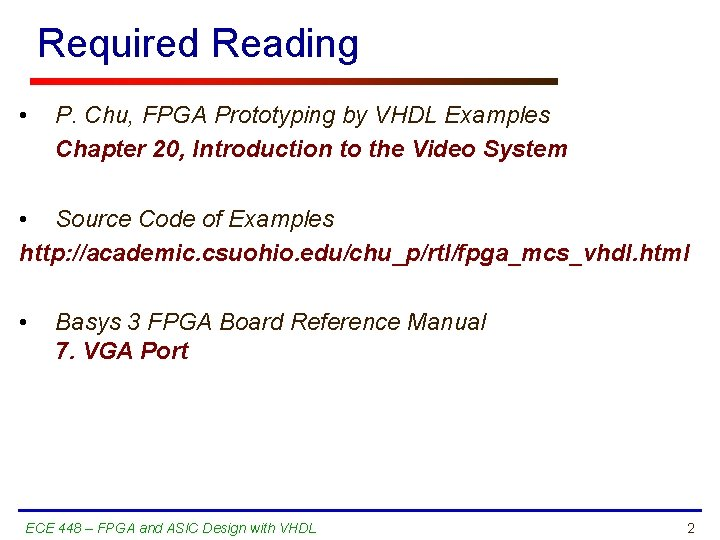Required Reading • P. Chu, FPGA Prototyping by VHDL Examples Chapter 20, Introduction to the Video System • Source Code of Examples http: //academic. csuohio. edu/chu_p/rtl/fpga_mcs_vhdl. html • Basys 3 FPGA Board Reference Manual 7. VGA Port ECE 448 – FPGA and ASIC Design with VHDL 2Basics ECE 448 – FPGA and ASIC Design with VHDL 3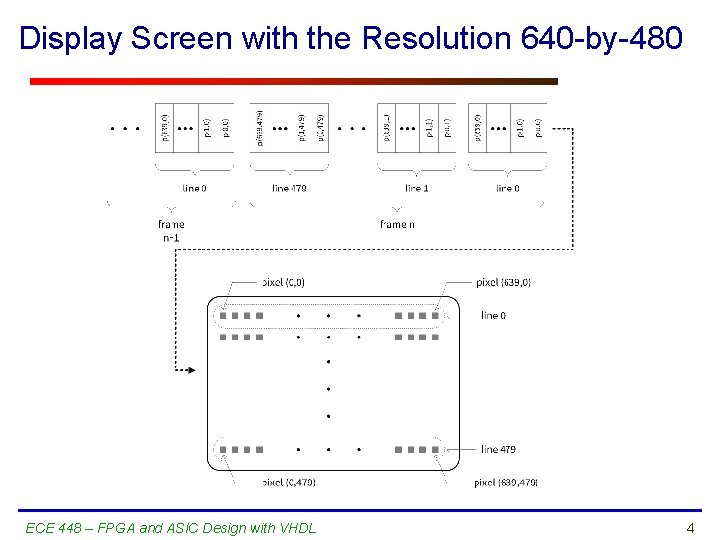Display Screen with the Resolution 640 -by-480 ECE 448 – FPGA and ASIC Design with VHDL 4VGA – Video Graphics Array • Video display standard introduced in the late 1980’s • Widely supported by PC graphics hardware and monitors • Used initially with the CRT (cathode ray tube) monitors • Later adopted for LCD (liquid-crystal display) monitors as well ECE 448 – FPGA and ASIC Design with VHDL 5VGA – Characteristic Features • • Resolution: 640 x 480 Typical Refresh Rate: 60 Hz (frames / second) RGB: Red, Green and Blue analog signals Color depth: number of bits used to encode a color of each pixel § True color mode: 24 bits § Basic color mode: 3 bits § Monochrome: 1 bit ECE 448 – FPGA and ASIC Design with VHDL 6Three-bit VGA Color Combinations ECE 448 – FPGA and ASIC Design with VHDL 7Operation of a CRT monitor ECE 448 – FPGA and ASIC Design with VHDL 8CRT Monitor – Conceptual Diagram ECE 448 – FPGA and ASIC Design with VHDL 9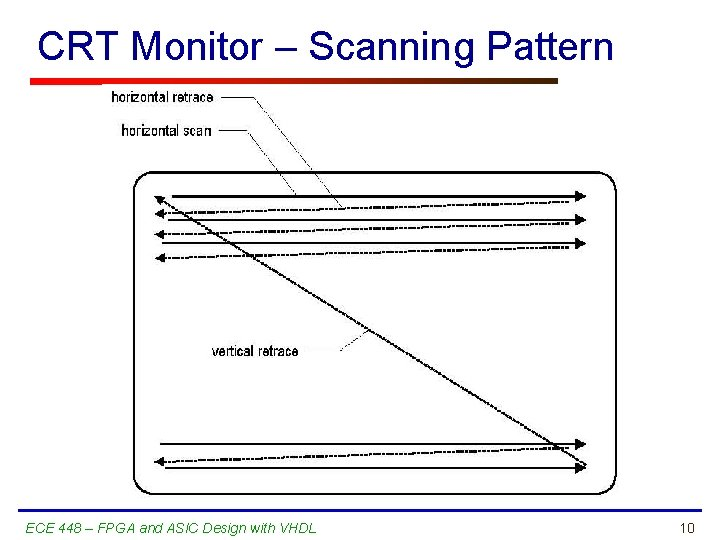CRT Monitor – Scanning Pattern ECE 448 – FPGA and ASIC Design with VHDL 10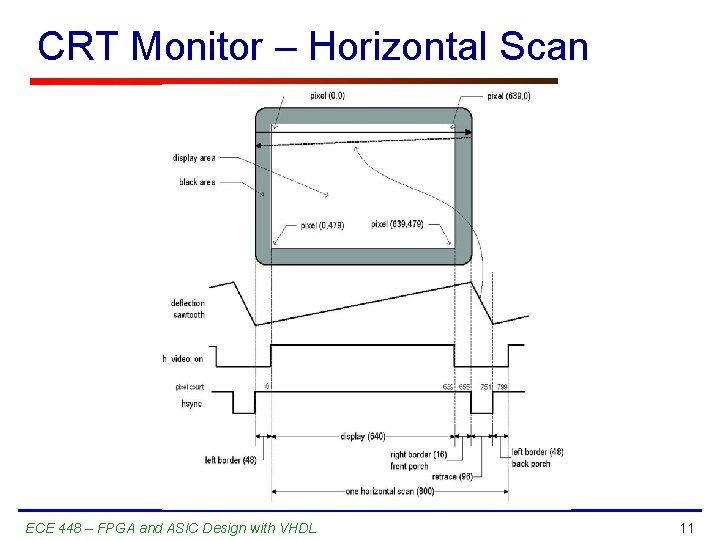CRT Monitor – Horizontal Scan ECE 448 – FPGA and ASIC Design with VHDL 11VGA Controller ECE 448 – FPGA and ASIC Design with VHDL 12VGA Controller – Simplified View ECE 448 – FPGA and ASIC Design with VHDL 13VGA Synchronization ECE 448 – FPGA and ASIC Design with VHDL 14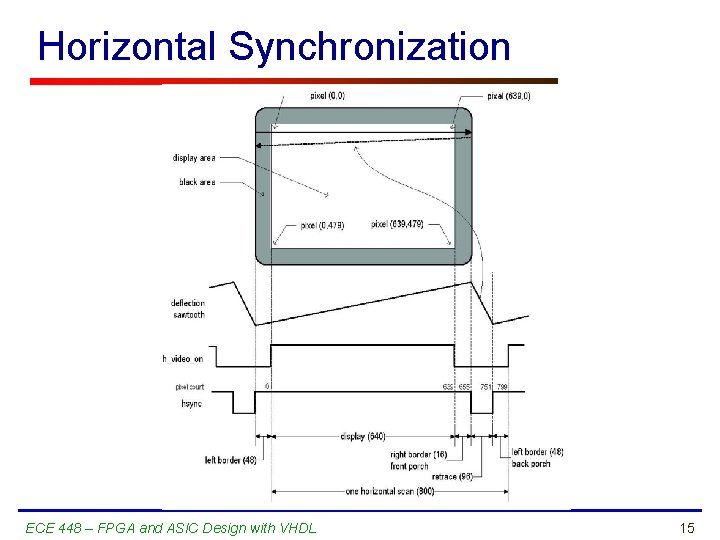Horizontal Synchronization ECE 448 – FPGA and ASIC Design with VHDL 15Four regions of hsync • • Display: 0. . 639, width = 640 Right border (front porch): 640. . 655, width = 16 Retrace (horizontal flyback): 656. . 751, width=96 Left border (back porch): 752. . 799, width=48 ECE 448 – FPGA and ASIC Design with VHDL 16Vertical Synchronization ECE 448 – FPGA and ASIC Design with VHDL 17Four regions of vsync • • Display: 0. . 479, width = 480 lines Bottom border (front porch): 480. . 489, width = 10 Retrace (vertical flyback): 490. . 491, width=2 Top border (back porch): 491. . 524, width=33 ECE 448 – FPGA and ASIC Design with VHDL 18Pixel Rate • p: the number of pixels in a horizontal scan line p = 800 pixels/line • l: the number of horizontal lines in a screen l = 525 lines/screen • s: the number of screens per second (refresh rate) s = 60 screens/second Pixel Clock Rate = p ・ l ・ s = 25 Mpixels/second Pixel Data Rate = 640・ 480・ 60 = 18. 4 Mpixels/second ECE 448 – FPGA and ASIC Design with VHDL 19VHDL Code of VGA Sync ECE 448 – FPGA and ASIC Design with VHDL 20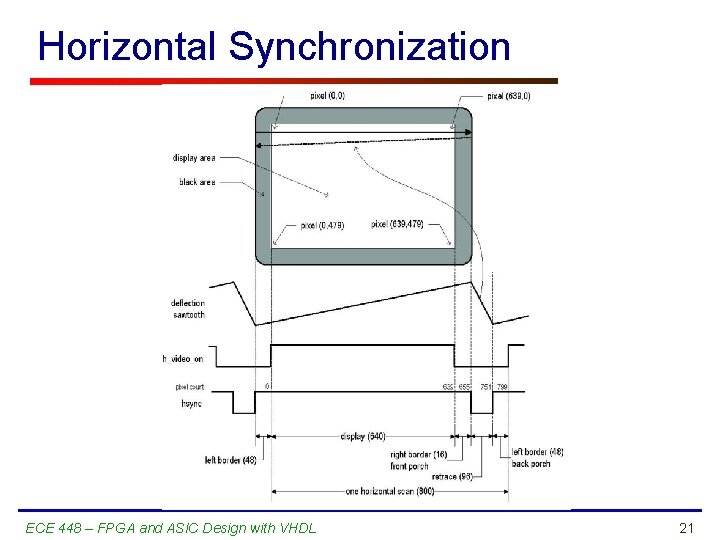Horizontal Synchronization ECE 448 – FPGA and ASIC Design with VHDL 21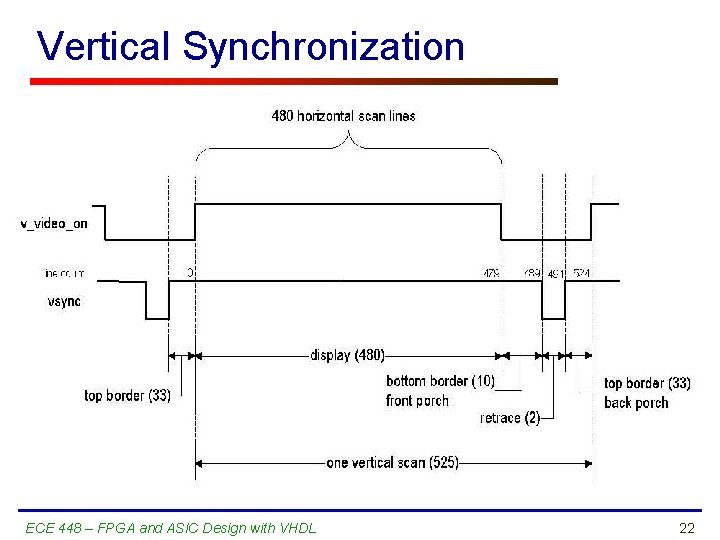Vertical Synchronization ECE 448 – FPGA and ASIC Design with VHDL 22VHDL Code of VGA Sync (1) entity vga_sync_demo is generic(CD : integer : = 12); -- color depth port( clk, reset : in std_logic; -- stream input vga_si_rgb : in std_logic_vector(CD - 1 downto 0); -- to vga monitor hsync : out std_logic; vsync : out std_logic; rgb : out std_logic_vector(CD - 1 downto 0); -- frame counter output hc, vc : out std_logic_vector(10 downto 0) ); end vga_sync_demo; ECE 448 – FPGA and ASIC Design with VHDL 23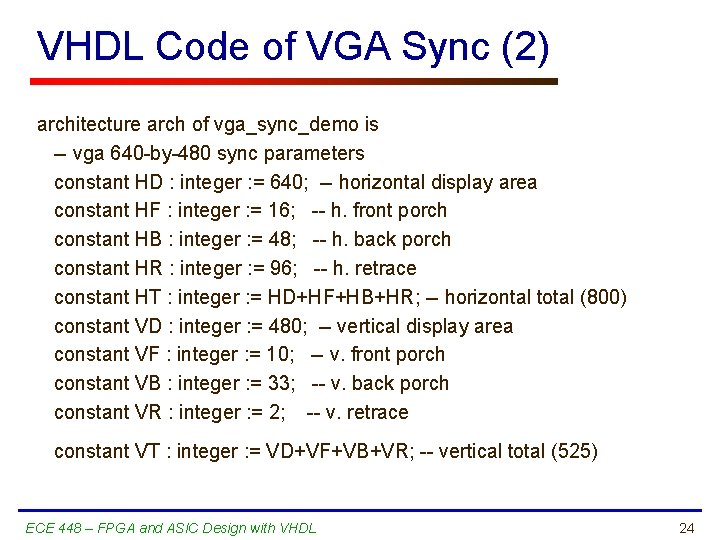VHDL Code of VGA Sync (2) architecture arch of vga_sync_demo is -- vga 640 -by-480 sync parameters constant HD : integer : = 640; -- horizontal display area constant HF : integer : = 16; -- h. front porch constant HB : integer : = 48; -- h. back porch constant HR : integer : = 96; -- h. retrace constant HT : integer : = HD+HF+HB+HR; -- horizontal total (800) constant VD : integer : = 480; -- vertical display area constant VF : integer : = 10; -- v. front porch constant VB : integer : = 33; -- v. back porch constant VR : integer : = 2; -- v. retrace constant VT : integer : = VD+VF+VB+VR; -- vertical total (525) ECE 448 – FPGA and ASIC Design with VHDL 24VHDL Code of VGA Sync (3) -- sync counter and signals signal x, y : unsigned(10 downto 0); signal hcount : std_logic_vector(10 downto 0); signal vcount : std_logic_vector(10 downto 0); signal hsync_i : std_logic; signal video_on_i : std_logic; signal q_reg : unsigned(1 downto 0); signal tick_25 M : std_logic; ECE 448 – FPGA and ASIC Design with VHDL 25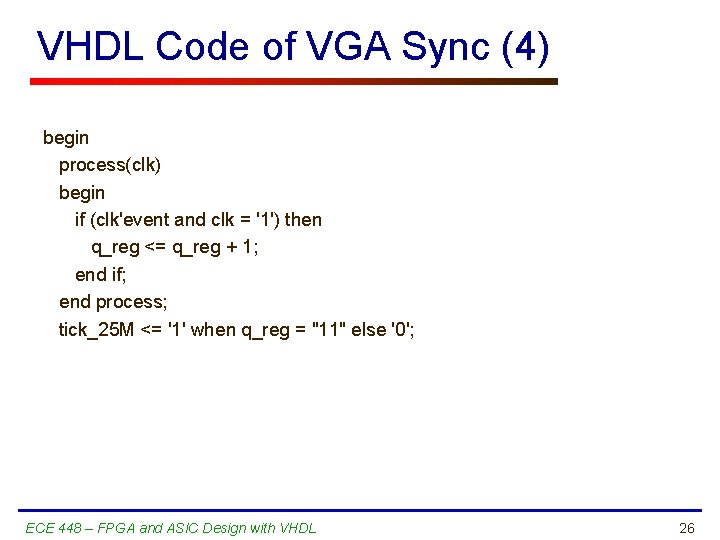VHDL Code of VGA Sync (4) begin process(clk) begin if (clk'event and clk = '1') then q_reg <= q_reg + 1; end if; end process; tick_25 M <= '1' when q_reg = "11" else '0'; ECE 448 – FPGA and ASIC Design with VHDL 26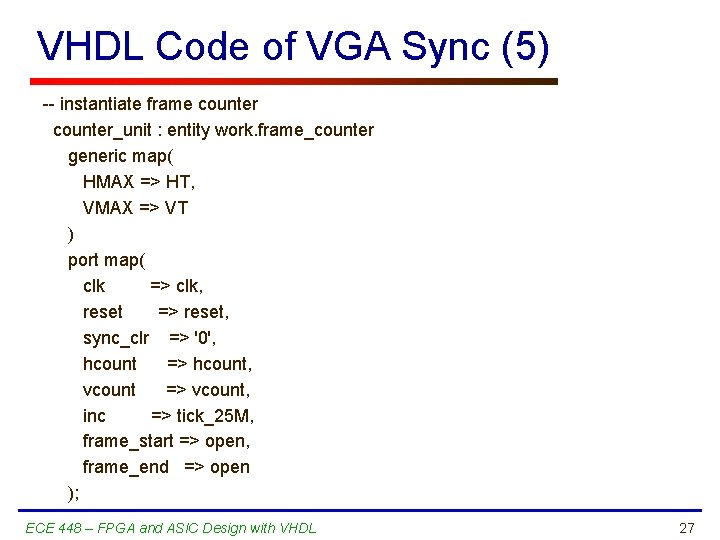VHDL Code of VGA Sync (5) -- instantiate frame counter_unit : entity work. frame_counter generic map( HMAX => HT, VMAX => VT ) port map( clk => clk, reset => reset, sync_clr => '0', hcount => hcount, vcount => vcount, inc => tick_25 M, frame_start => open, frame_end => open ); ECE 448 – FPGA and ASIC Design with VHDL 27VHDL Code of VGA Sync (6) x <= unsigned(hcount); y <= unsigned(vcount); hc <= hcount; vc <= vcount; -- horizontal sync decoding hsync_i <= '0' when (x>=(HD+HF)) and (x<=(HD+HF+HR-1)) else '1'; -- vertical sync decoding vsync_i <= '0' when (y>=(VD+VF)) and (y<=(VD+VF+VR-1)) else '1'; -- display on/off video_on_i <= '1' when (x < HD) and (y < VD) else '0'; ECE 448 – FPGA and ASIC Design with VHDL 28VHDL Code of VGA Sync (7) -- buffered output to vga monitor process(clk) begin if (clk'event and clk = '1') then vsync <= vsync_i; hsync <= hsync_i; if (video_on_i = '1') then rgb <= vga_si_rgb; else rgb <= (others => '0'); -- black when display off end if; end process; end arch; ECE 448 – FPGA and ASIC Design with VHDL 29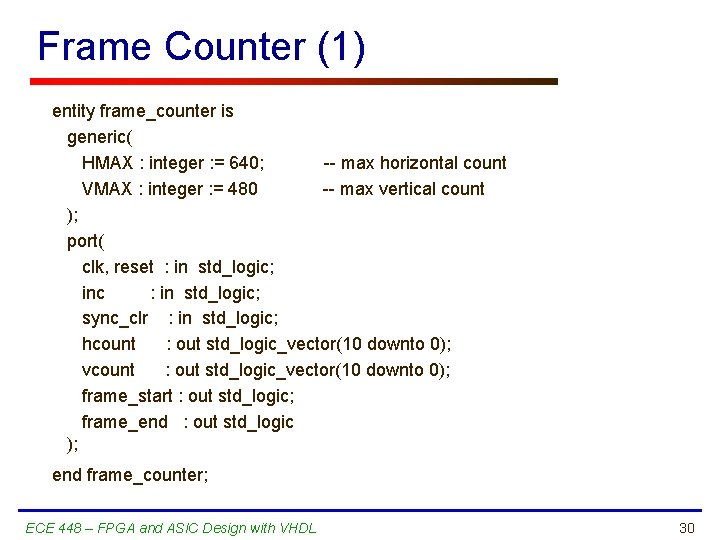Frame Counter (1) entity frame_counter is generic( HMAX : integer : = 640; -- max horizontal count VMAX : integer : = 480 -- max vertical count ); port( clk, reset : in std_logic; inc : in std_logic; sync_clr : in std_logic; hcount : out std_logic_vector(10 downto 0); vcount : out std_logic_vector(10 downto 0); frame_start : out std_logic; frame_end : out std_logic ); end frame_counter; ECE 448 – FPGA and ASIC Design with VHDL 30Frame Counter (2) architecture arch of frame_counter is signal hc_reg, hc_next : unsigned(10 downto 0); signal vc_reg, vc_next : unsigned(10 downto 0); begin process(clk, reset) begin if reset = '1' then vc_reg <= (others => '0'); hc_reg <= (others => '0'); elsif (clk'event and clk = '1') then if (sync_clr = '1') then vc_reg <= (others => '0'); hc_reg <= (others => '0'); else vc_reg <= vc_next; hc_reg <= hc_next; end if; end process; ECE 448 – FPGA and ASIC Design with VHDL 31Frame Counter (3) -- next-state logic of horizontal counter process(hc_reg, inc) begin if (inc = '1') then if hc_reg = (HMAX - 1) then hc_next <= (others => '0'); else hc_next <= hc_reg + 1; end if; else hc_next <= hc_reg; end if; end process; ECE 448 – FPGA and ASIC Design with VHDL 32Frame Counter (4) -- next-state logic of vertical counter process(vc_reg, hc_reg, inc) begin if (inc = '1') and hc_reg = (HMAX - 1) then if vc_reg = (VMAX - 1) then vc_next <= (others => '0'); else vc_next <= vc_reg + 1; end if; else vc_next <= vc_reg; end if; end process; ECE 448 – FPGA and ASIC Design with VHDL 33Frame Counter (5) -- output hcount <= std_logic_vector(hc_reg); vcount <= std_logic_vector(vc_reg); frame_start <= '1' when vc_reg = 0 and hc_reg = 0 else '0'; frame_end <= '1' when vc_reg = (VMAX - 1) and hc_reg = (HMAX - 1) else '0'; end arch; ECE 448 – FPGA and ASIC Design with VHDL 34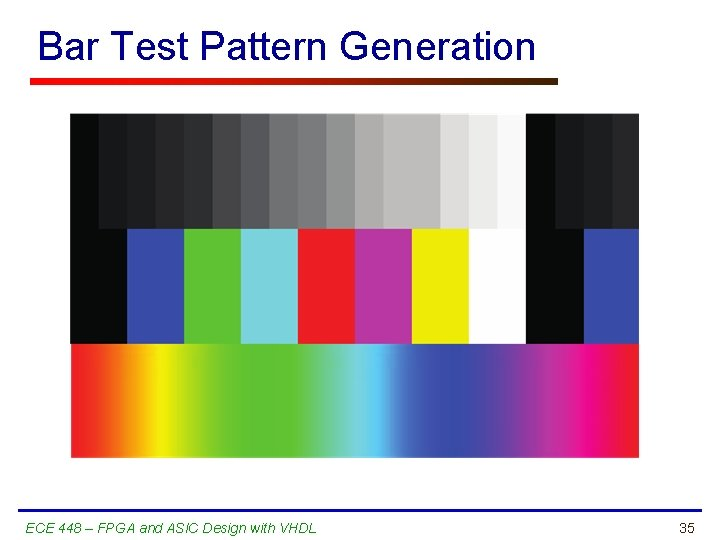Bar Test Pattern Generation ECE 448 – FPGA and ASIC Design with VHDL 35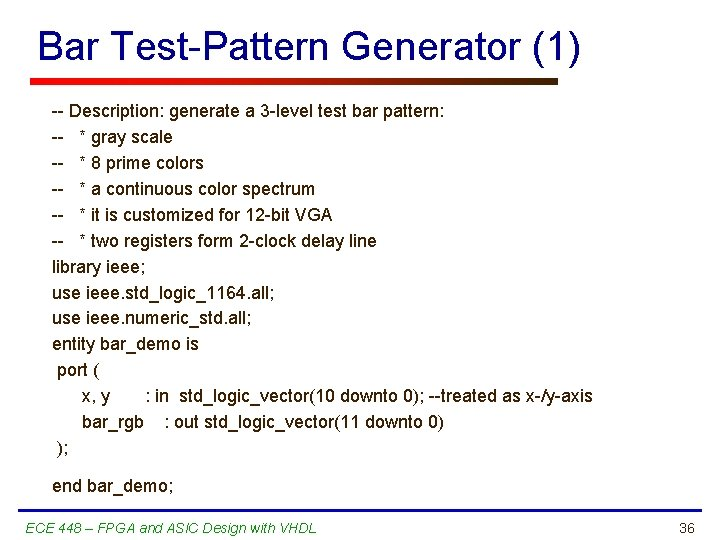Bar Test-Pattern Generator (1) -- Description: generate a 3 -level test bar pattern: -- * gray scale -- * 8 prime colors -- * a continuous color spectrum -- * it is customized for 12 -bit VGA -- * two registers form 2 -clock delay line library ieee; use ieee. std_logic_1164. all; use ieee. numeric_std. all; entity bar_demo is port ( x, y : in std_logic_vector(10 downto 0); --treated as x-/y-axis bar_rgb : out std_logic_vector(11 downto 0) ); end bar_demo; ECE 448 – FPGA and ASIC Design with VHDL 36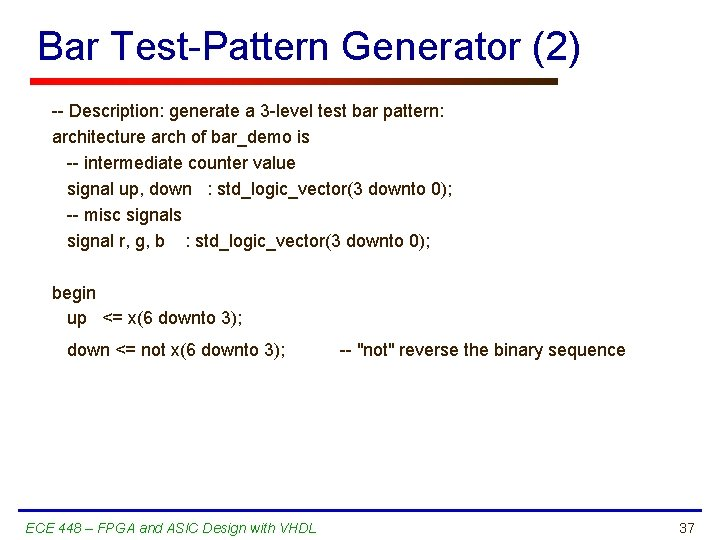Bar Test-Pattern Generator (2) -- Description: generate a 3 -level test bar pattern: architecture arch of bar_demo is -- intermediate counter value signal up, down : std_logic_vector(3 downto 0); -- misc signals signal r, g, b : std_logic_vector(3 downto 0); begin up <= x(6 downto 3); down <= not x(6 downto 3); ECE 448 – FPGA and ASIC Design with VHDL -- "not" reverse the binary sequence 37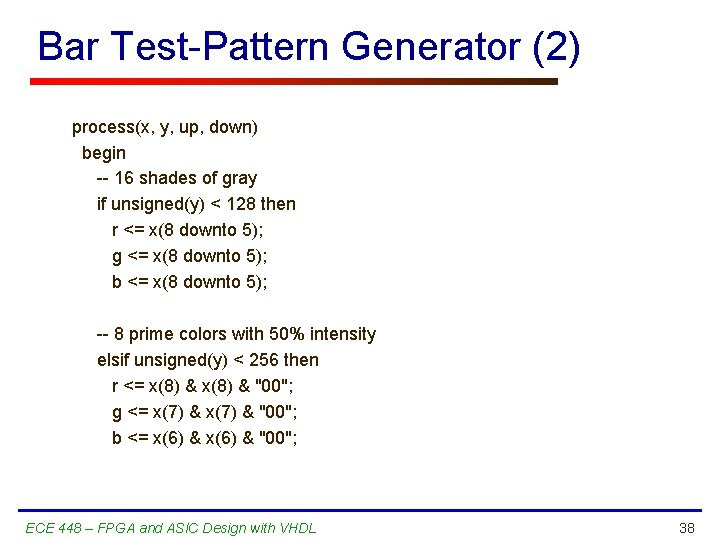Bar Test-Pattern Generator (2) process(x, y, up, down) begin -- 16 shades of gray if unsigned(y) < 128 then r <= x(8 downto 5); g <= x(8 downto 5); b <= x(8 downto 5); -- 8 prime colors with 50% intensity elsif unsigned(y) < 256 then r <= x(8) & "00"; g <= x(7) & "00"; b <= x(6) & "00"; ECE 448 – FPGA and ASIC Design with VHDL 38Bar Test Pattern Generation ECE 448 – FPGA and ASIC Design with VHDL 39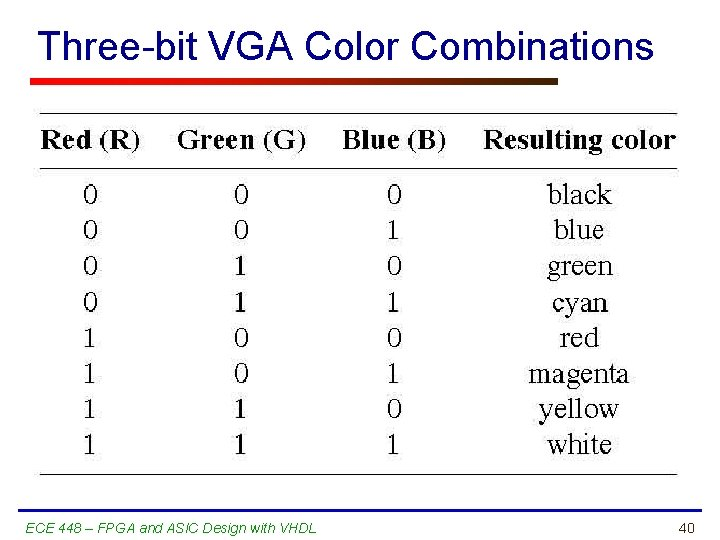Three-bit VGA Color Combinations ECE 448 – FPGA and ASIC Design with VHDL 40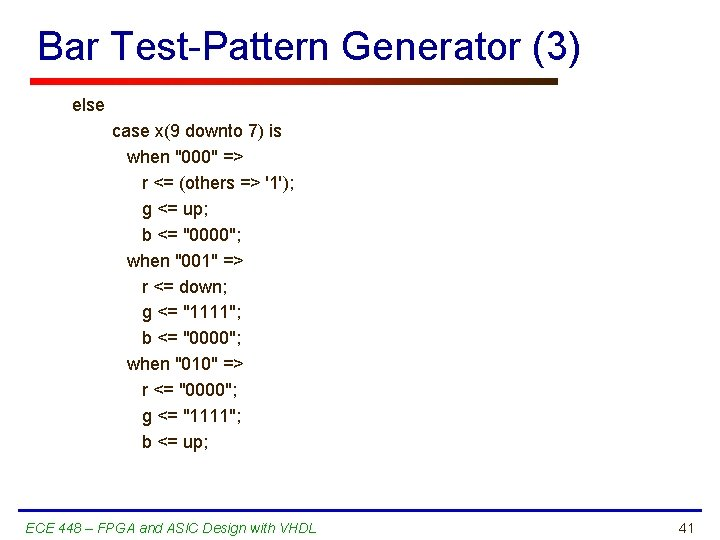Bar Test-Pattern Generator (3) else case x(9 downto 7) is when "000" => r <= (others => '1'); g <= up; b <= "0000"; when "001" => r <= down; g <= "1111"; b <= "0000"; when "010" => r <= "0000"; g <= "1111"; b <= up; ECE 448 – FPGA and ASIC Design with VHDL 41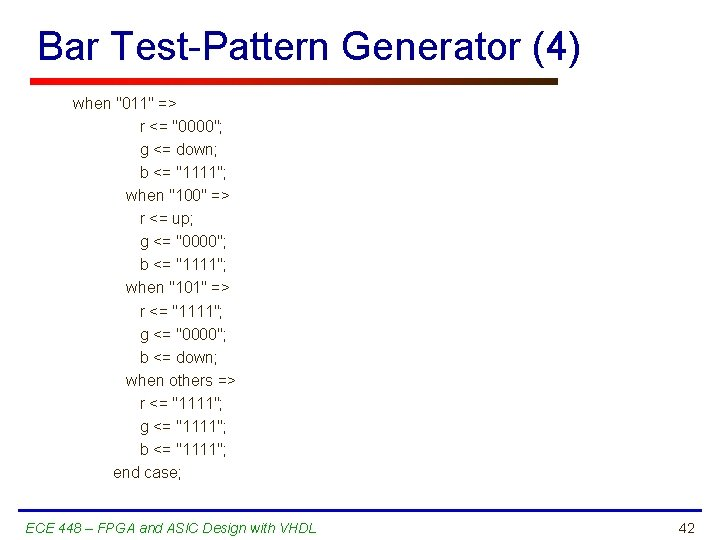Bar Test-Pattern Generator (4) when "011" => r <= "0000"; g <= down; b <= "1111"; when "100" => r <= up; g <= "0000"; b <= "1111"; when "101" => r <= "1111"; g <= "0000"; b <= down; when others => r <= "1111"; g <= "1111"; b <= "1111"; end case; ECE 448 – FPGA and ASIC Design with VHDL 42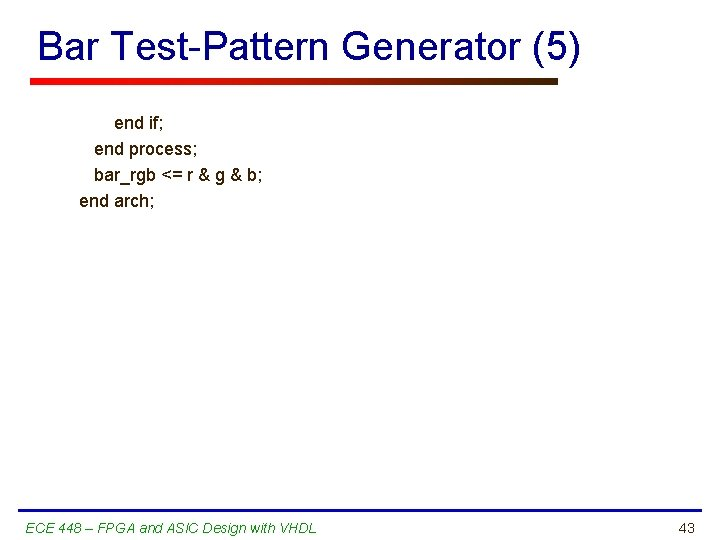Bar Test-Pattern Generator (5) end if; end process; bar_rgb <= r & g & b; end arch; ECE 448 – FPGA and ASIC Design with VHDL 43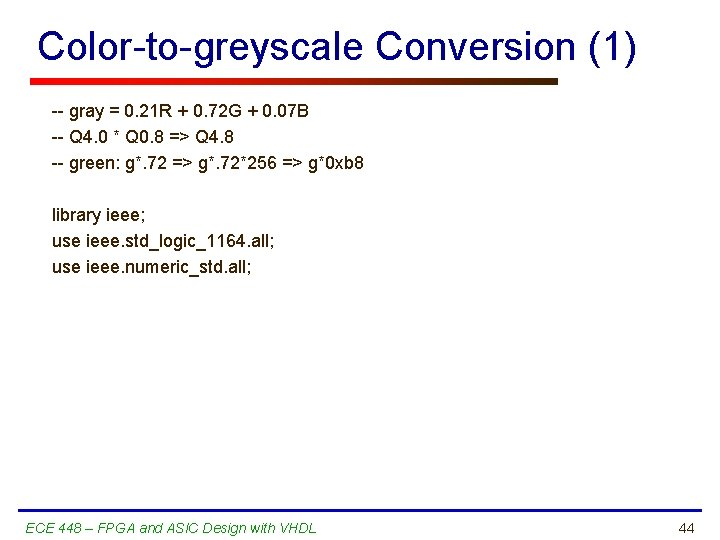Color-to-greyscale Conversion (1) -- gray = 0. 21 R + 0. 72 G + 0. 07 B -- Q 4. 0 * Q 0. 8 => Q 4. 8 -- green: g*. 72 => g*. 72*256 => g*0 xb 8 library ieee; use ieee. std_logic_1164. all; use ieee. numeric_std. all; ECE 448 – FPGA and ASIC Design with VHDL 44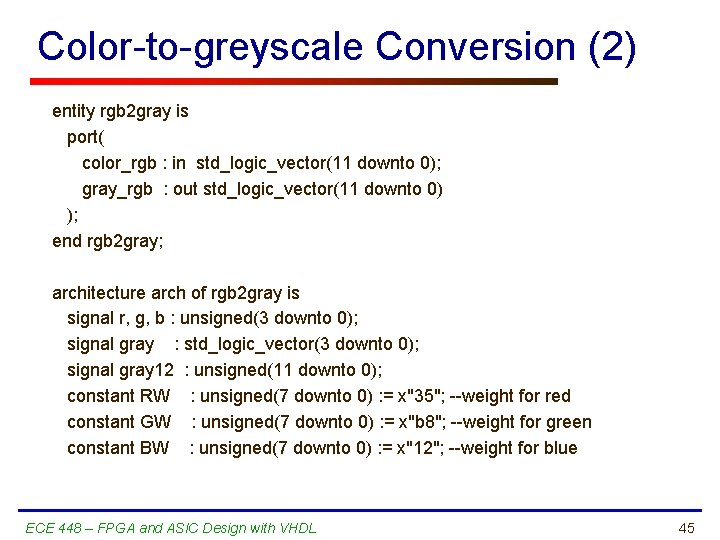Color-to-greyscale Conversion (2) entity rgb 2 gray is port( color_rgb : in std_logic_vector(11 downto 0); gray_rgb : out std_logic_vector(11 downto 0) ); end rgb 2 gray; architecture arch of rgb 2 gray is signal r, g, b : unsigned(3 downto 0); signal gray : std_logic_vector(3 downto 0); signal gray 12 : unsigned(11 downto 0); constant RW : unsigned(7 downto 0) : = x"35"; --weight for red constant GW : unsigned(7 downto 0) : = x"b 8"; --weight for green constant BW : unsigned(7 downto 0) : = x"12"; --weight for blue ECE 448 – FPGA and ASIC Design with VHDL 45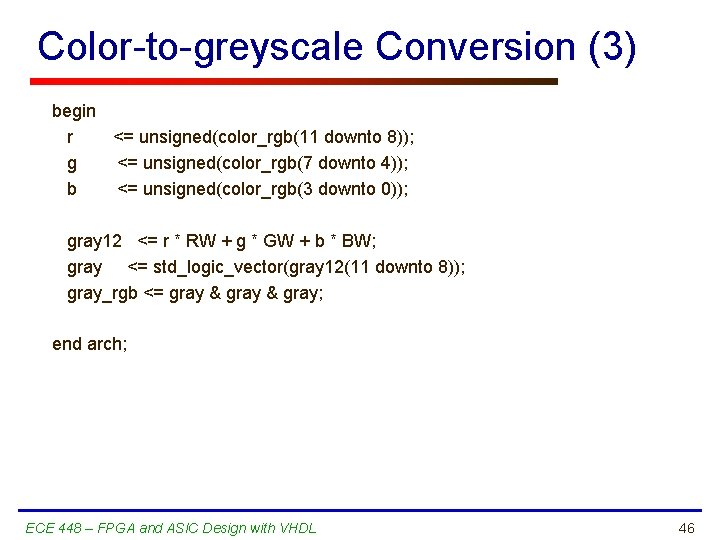Color-to-greyscale Conversion (3) begin r <= unsigned(color_rgb(11 downto 8)); g <= unsigned(color_rgb(7 downto 4)); b <= unsigned(color_rgb(3 downto 0)); gray 12 <= r * RW + g * GW + b * BW; gray <= std_logic_vector(gray 12(11 downto 8)); gray_rgb <= gray & gray; end arch; ECE 448 – FPGA and ASIC Design with VHDL 46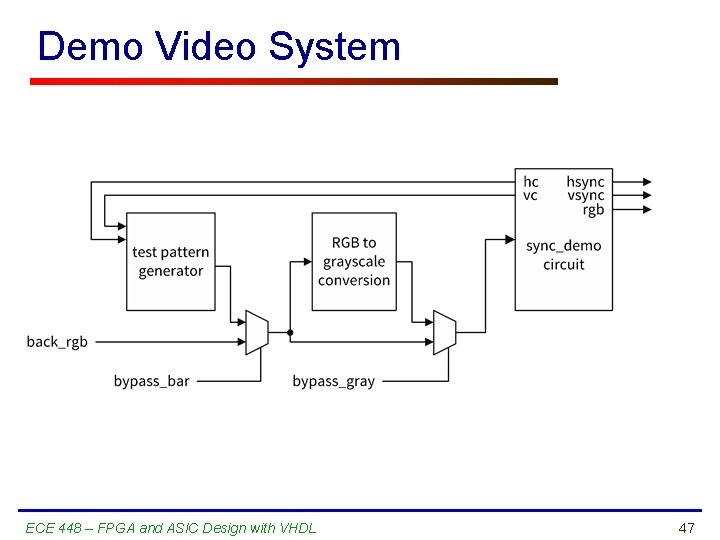Demo Video System ECE 448 – FPGA and ASIC Design with VHDL 47Demo Video System (1) library ieee; use ieee. std_logic_1164. all; use ieee. numeric_std. all; entity vga_demo is generic( CD : integer : = 12 -- color depth ); port( clk : in std_logic; -- switch control sw : in std_logic_vector(13 downto 0); -- to vga monitor hsync : out std_logic; vsync : out std_logic; rgb : out std_logic_vector(CD - 1 downto 0) ); end vga_demo; ECE 448 – FPGA and ASIC Design with VHDL 48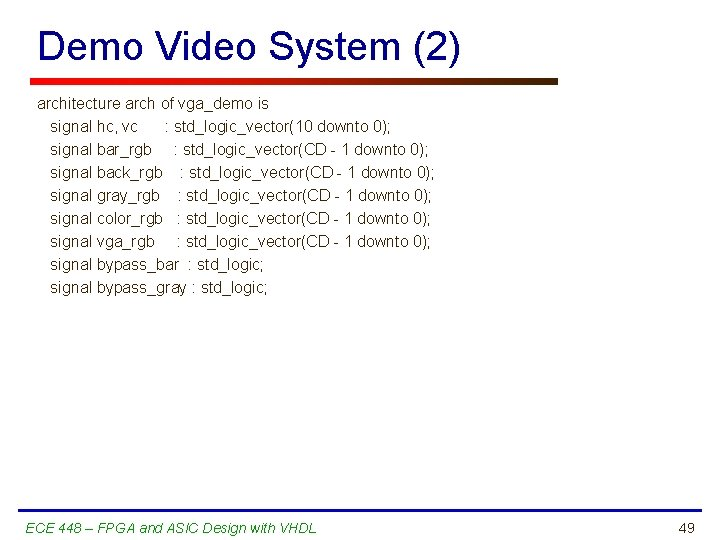Demo Video System (2) architecture arch of vga_demo is signal hc, vc : std_logic_vector(10 downto 0); signal bar_rgb : std_logic_vector(CD - 1 downto 0); signal back_rgb : std_logic_vector(CD - 1 downto 0); signal gray_rgb : std_logic_vector(CD - 1 downto 0); signal color_rgb : std_logic_vector(CD - 1 downto 0); signal vga_rgb : std_logic_vector(CD - 1 downto 0); signal bypass_bar : std_logic; signal bypass_gray : std_logic; ECE 448 – FPGA and ASIC Design with VHDL 49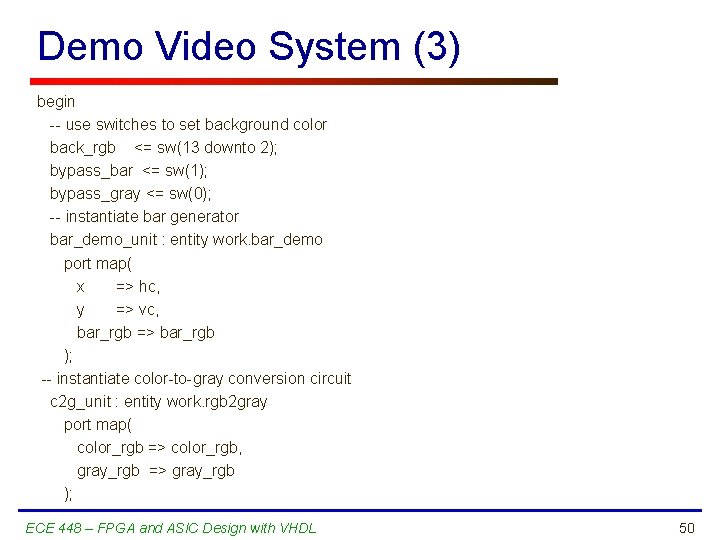Demo Video System (3) begin -- use switches to set background color back_rgb <= sw(13 downto 2); bypass_bar <= sw(1); bypass_gray <= sw(0); -- instantiate bar generator bar_demo_unit : entity work. bar_demo port map( x => hc, y => vc, bar_rgb => bar_rgb ); -- instantiate color-to-gray conversion circuit c 2 g_unit : entity work. rgb 2 gray port map( color_rgb => color_rgb, gray_rgb => gray_rgb ); ECE 448 – FPGA and ASIC Design with VHDL 50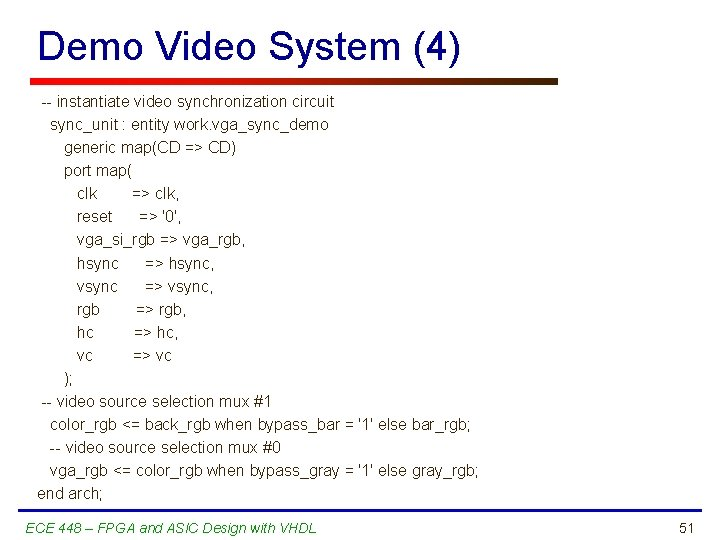Demo Video System (4) -- instantiate video synchronization circuit sync_unit : entity work. vga_sync_demo generic map(CD => CD) port map( clk => clk, reset => '0', vga_si_rgb => vga_rgb, hsync => hsync, vsync => vsync, rgb => rgb, hc => hc, vc => vc ); -- video source selection mux #1 color_rgb <= back_rgb when bypass_bar = '1' else bar_rgb; -- video source selection mux #0 vga_rgb <= color_rgb when bypass_gray = '1' else gray_rgb; end arch; ECE 448 – FPGA and ASIC Design with VHDL 51